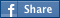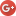Help

# Mathematical notation

warning: Creating default object from empty value in /var/www/vhosts/sayforward.com/subdomains/recorder/httpdocs/modules/taxonomy/taxonomy.pages.inc on line 33.

## 150 Gigapixel Sky Image Contains 1 Billion Stars

The Bad Astronomer writes "Astronomers have used two big telescopes to create an infrared survey of the Milky Way that is the largest of its kind: the resulting image has an incredible 150,000 megapixels containing over a billion stars. Something that large is difficult to use, so they also made a pan-and-zoom version online which should keep you occupied for quite some time. These data will be used to better understand star formation in our Milky Way, and how far more distant galaxies and quasars behave."

The interactive image is powered by IIPImage which happens to be Free Software and is cool in its own right (right click the image to get help — it has a full set of keybindings for navigation).Read more of this story at Slashdot.

## Processing Arithmetic Expressions with the Shunting-Yard Algorithm

In game development (or programming in general) it’s not uncommon to have a situation where you’d like to let a user enter an arithmetic formula that your code parses and evaluates. For example, in a shader you might like to have an annotation that specifies how a parameter is to...

## Knuth for Programmers: Sums and Products

(Here's an article I started writing but never finished. I'm never going to finish it, most likely - I've returned the book to the library, but I thought it still might have some value for someone.)

A friend recommended I read Knuth. As I slog through it I discover a lot of it is written in "math" - a language I haven't spoken in over twenty years. When I look at code, I can understand it fairly easily - but when I look at summation notation, it takes a while to parse.

So, that's weird. "The Art of Programming" is written for mathematicians, not programmers.

I discovered the best way to parse it to make sure I really grok it is to rewrite it in code. And I figured others might benefit from the results of my exercises. So, without further ado, the figures from Art of Computer Programming Section 1.2.3 - Sums and Produts.

In this C#-like pseudocode, I'll use 'integer' for an honest-to-God integer that could approach infinity and be well beyond the capacity for a computer to store, 'real' for an honest-to-God real of infinite precision.

No guarantee any of this is 'right', btw. And the true value for me was in doing the work myself - not in being able to look at and understand this code later.

(1)

delegate real aFn(integer j);
// here we're expected to pass a function for a that simply indexes into an
// array

real sum( aFn a, integer n ) // sum elements of a from 0 to n
{
real accumulator=0;
for( int j=1; j<=n; j++ )  // Knuth's a array starts at 1
{
accumulator+=j;
}
return accumulator;

It's no wonder Knuth wrote in math - it's much more compact. For the next one, we have to imagine our magic computer that can store an infinite real can also iterate infinitely and return.

(2)

delegate real aFn(int j); // pass a function that indexes into array
delegate bool RFn(int j);  // R predicate

// here a is a function that returns an element of an array
real sum( aFn a, RFn R )
{
real accumulator=0;
for( integer j=0;;j++ )    // magic computer that iterates infinitely
{
if( R(j) )
accumulator+= a(j);
}

for( integer j=-1;;j-- )    // don't forget the negative integers
{
if( R(j) )
accumulator+= a(j);
}
return accumulator;
}

To make sure my code was correct, I wrote it using floats and ints to iterate from int.MinValue to int.MaxValue. Took a long time to execute.

Hey, and I just realized that (1) could also be written:

delegate real AFn(integer j);
real sum( AFn a, integer n )
{
return sum( a, delegate bool one_to_n(integer j) { return 1<=j && j<=n; });

(2.5)

sum( a, delegate(int j) { return j>=1; } )  // == a + a + a ...

(3)

// function prototype for limit
delegate real aFn( integer j );
delegate real RFn( integer j );
delegate real sumFn( aFn a, RFn r );
real limit( real nApproaches, sumFn summed, int lowJ, int highJ );

sum( a, R ) == limit( INFINITY, sum( a, R, 0, n )) +
limit( INFINITY, sum( a, R, -n, -1));

(4)

delegate real a(integer); // index into array a
delegate real b(integer); // index into array b
delegate bool R(integer); // is this element of a one we want to sum?
delegate bool S(integer); // is this element of b one we want to sum?

sum( a, R ) * sum( b, S) ==
sum( delegate( int i )
{
return sum( delegate( int j )
{ return a(i)*b(j); },
S );
}, R );

is true

(5)

The first bit of (5) is trivial - the second ... "the reader should study this example carefully."

// sum of permutation of range
delegate real aFn(int j); // pass a function that indexes into array
delegate bool RFn(int j);  // R predicate
delegate int pFn(int j);  // p permutation

real sum( aFn a, RFn R, pFn p )
{

real accumulator=0;
for( integer j=0;;j++ )    // magic computer that iterates infinitely
{
if( R( p(j) ) )
accumulator+= a( p(j) );
}

for( integer j=-1;;j-- )    // don't forget the negative integers
{
if( R( p(j) ) )
accumulator+= a( p(j) );
}
return accumulator;
}

sum( a, R ) == sum( a, R, p );

It's obvious once you can read it.

(6)

sum( a, n ) == sum( a, delegate(int) { return (1<=(j-1)) && ((j-1)<=n);} )
== sum( a, delegate(int) { return (2<=j) &&(j<=(n+1);},
delegate(int) { return j-1; })

An example helps me grok 6.

aFn a = delegate(integer j) { return j; }
n = 2

(1+2) == ((1+1-1)+(2+1-1)) == (2-1)+(3-1)

Makes perfect sense. I hope that's going to be as important as Knuth makes it out to be.

(7) Yikes. After taking a stab I realized I don't know if a sub-i sub-j means a two-dimensional array or if we're supposed to multiply i and j together or what. Guess I'll google 'interchanging order of summation'.  Ah. Two-dimensional array.

delegate real a2dFn(integer i, integer j);

sum( delegate(integer i)
{ return sum( a, S, delegate(integer j) { return a2dFn( i, j ); } ); }, R ) ==

sum( delegate(integer j)
{ return sum( a, R, delegate(integer i) { return a2dFn( i, j ); } ); }, S );

http://people.revoledu.com/kardi/tutorial/BasicMath/Sum/Double%20summation.htm

And I'm going to punt on 8 (which seems obvious), 9 (which seems like a worm can), and 10 - I may come back later.

(11)

sum( a, R ) + sum( a, S ) ==

sum( a, delegate(integer j) { return R(j) || S(j); }) +
sum( a, delegate(integer j) { return R(j) && S(j); });

I'm starting to think Knuth has Asperger's, by the way.

And, well, there it is. That's all I finished.